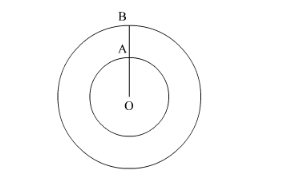# A circular park has a path of uniform width around it.Question:

A circular park has a path of uniform width around it. The difference between the outer and inner circumferences of the circular path is 132 m. Its width is

(a) 20 m

(b) 21 m

(c) 22 m

(d) 24 m

Solution:Let OA = r be the radius of the inner circle and OB = r be the radius of the outer circle.

Therefore, circumference of the inner circle $=2 \pi r$ and circumference of the outer circle $=2 \pi r^{\prime}$

Here we have to find the width of the circular park that is we have to find $r^{\prime}-r$.

We have given the difference between the circumferences of outer circle and inner circle.

$\therefore 2 \pi r^{\prime}-2 \pi r=132$

$\therefore 2 \pi\left(r^{\prime}-r\right)=132$

Substituting $\pi=\frac{22}{7}$ we get,

$2 \times \frac{22}{7} \times\left(r^{\prime}-r\right)=132$

Now we will multiply both sides of the equation by $\frac{7}{44}$.

$\left(r^{\prime}-r\right)=132 \times \frac{7}{44}$

$\therefore\left(r^{\prime}-r\right)=3 \times 7$

$\therefore\left(r^{\prime}-r\right)=21$

Therefore, the width is $21 \mathrm{~m}$.

Hence the correct answer is option (b).